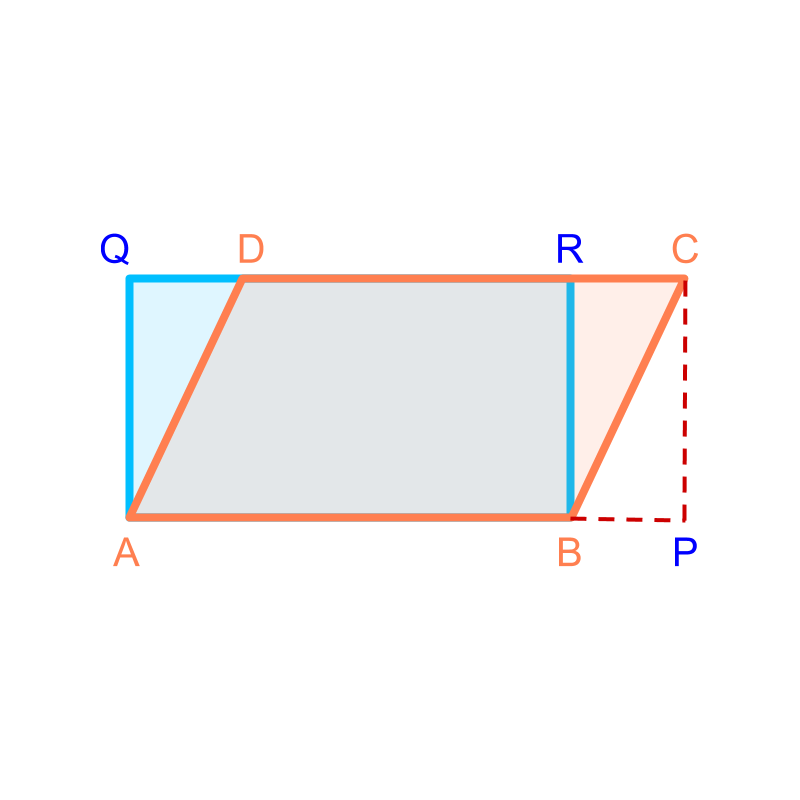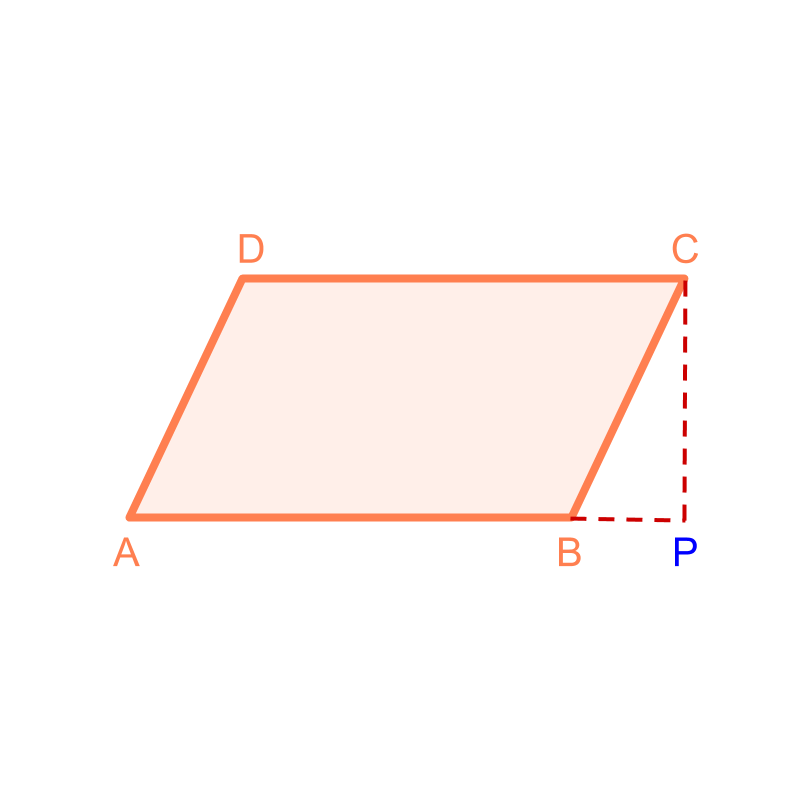maths > mensuration-high

Measurement by Equivalence

what you'll learn...

Overview

Measurement by Equivalence: Length, Area, or Volume can be measured by constructing an equivalent shape or object involving geometrical principles.consider a problemConsider the shape given in the figure. It is a parallelogram of base $b$$b$ centimeter and height $h$$h$ centimeter. To find the area of the parallelogram, the grid of unit squares is superimposed and the number of unit squared are counted. Since unit-squares will not fit exactly at the vertices, only approximate area can be computed.

equivalenceA rectangle $ABRQ$$A B R Q$ of length $b$$b$ cm and width $h$$h$ cm is shown in blue. Consider the region $△BRC$$\triangle B R C$ and the region $△AQD$$\triangle A Q D$. The area of these two triangles are equal.

The area of the parallelogram is sum of areas of the region $ABRD$$A B R D$ and $△BRC$$\triangle B R C$. This can be equivalently given as sum of areas of the region $ABRD$$A B R D$ and $△AQD$$\triangle A Q D$.

This implies that "area of the parallelogram equals the area of the rectangle".

summary

Measurement by Equivalence: Length, Area, or Volume can be measured by constructing an equivalent shape or object involving geometrical principles.This method suits best for

•  area of plane figures of curved sides or curved surfaces

•  volume of solid figures of curved edges or curved faces

Outline